Скачать презентацию QUANTITATIVE METHODS OF BUSINESS RESEARCH Class 6 Topic

Class 6 (2).ppt

• Количество слайдов: 74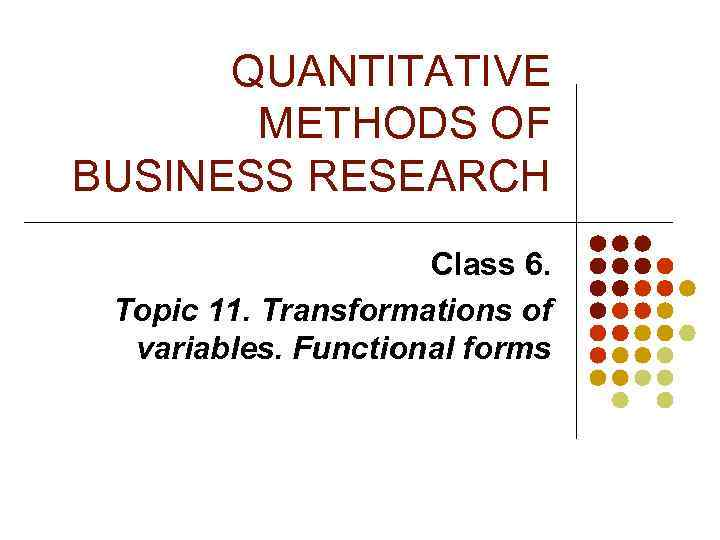QUANTITATIVE METHODS OF BUSINESS RESEARCH Class 6. Topic 11. Transformations of variables. Functional forms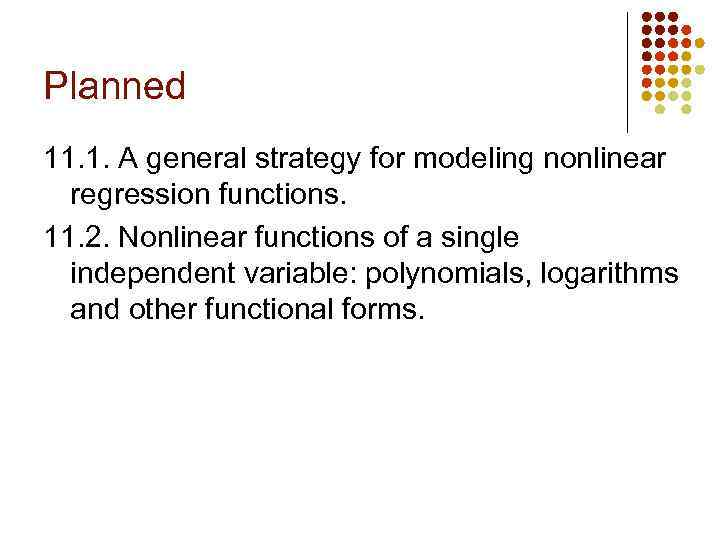Planned 11. 1. A general strategy for modeling nonlinear regression functions. 11. 2. Nonlinear functions of a single independent variable: polynomials, logarithms and other functional forms.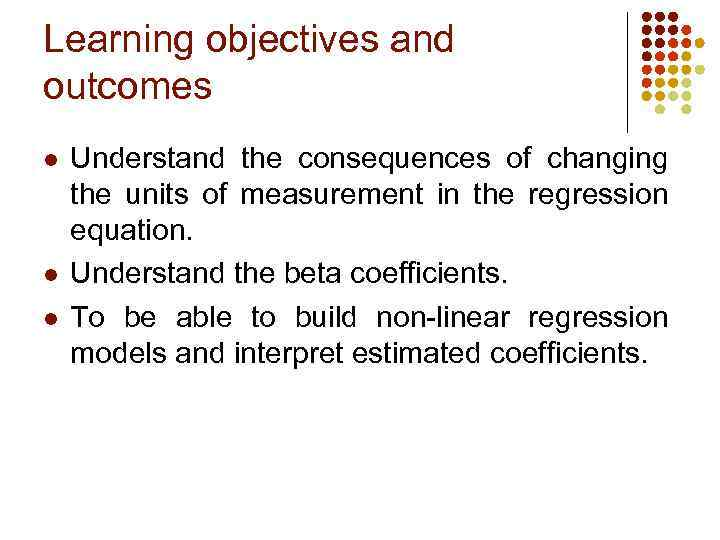Learning objectives and outcomes l l l Understand the consequences of changing the units of measurement in the regression equation. Understand the beta coefficients. To be able to build non-linear regression models and interpret estimated coefficients.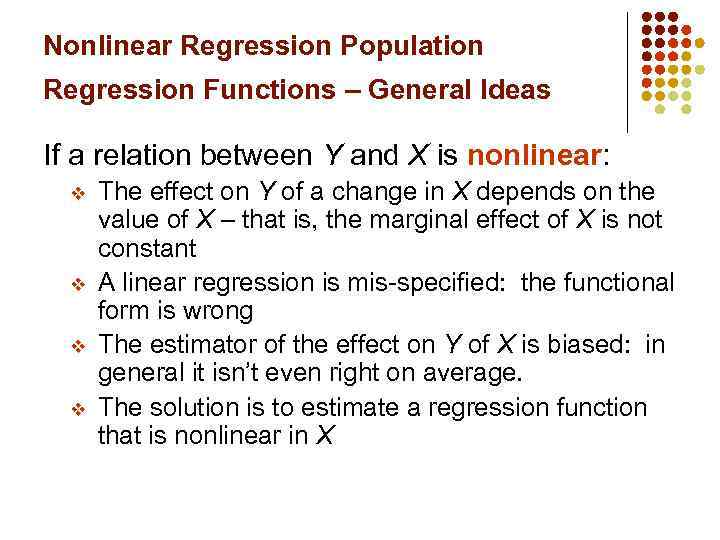Nonlinear Regression Population Regression Functions – General Ideas If a relation between Y and X is nonlinear: v v The effect on Y of a change in X depends on the value of X – that is, the marginal effect of X is not constant A linear regression is mis-specified: the functional form is wrong The estimator of the effect on Y of X is biased: in general it isn’t even right on average. The solution is to estimate a regression function that is nonlinear in X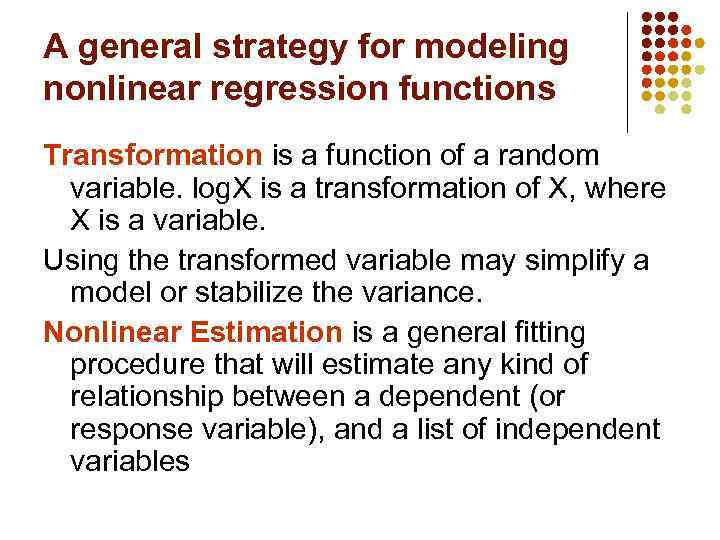A general strategy for modeling nonlinear regression functions Transformation is a function of a random variable. log. X is a transformation of X, where X is a variable. Using the transformed variable may simplify a model or stabilize the variance. Nonlinear Estimation is a general fitting procedure that will estimate any kind of relationship between a dependent (or response variable), and a list of independent variables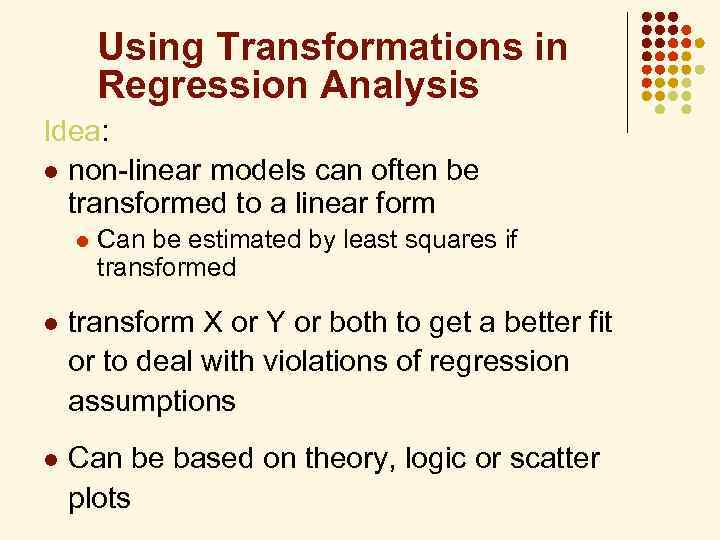Using Transformations in Regression Analysis Idea: l non-linear models can often be transformed to a linear form l Can be estimated by least squares if transformed l transform X or Y or both to get a better fit or to deal with violations of regression assumptions l Can be based on theory, logic or scatter plots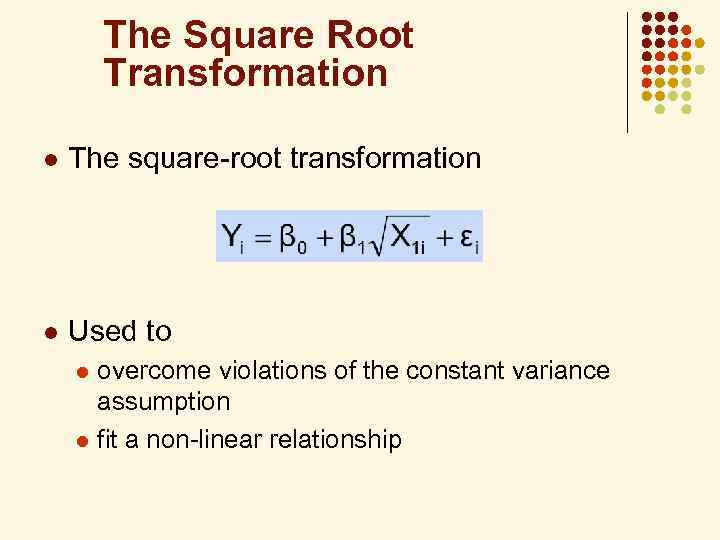The Square Root Transformation l The square-root transformation l Used to overcome violations of the constant variance assumption l fit a non-linear relationship l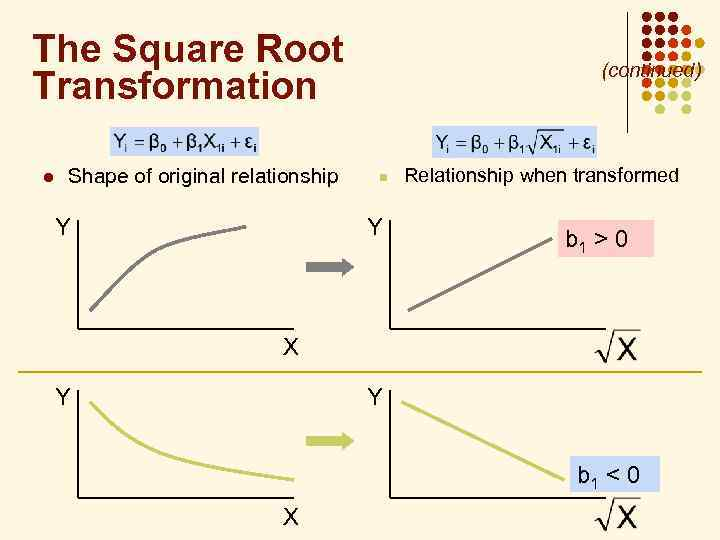The Square Root Transformation l Shape of original relationship Y (continued) n Y Relationship when transformed b 1 > 0 X Y Y b 1 < 0 X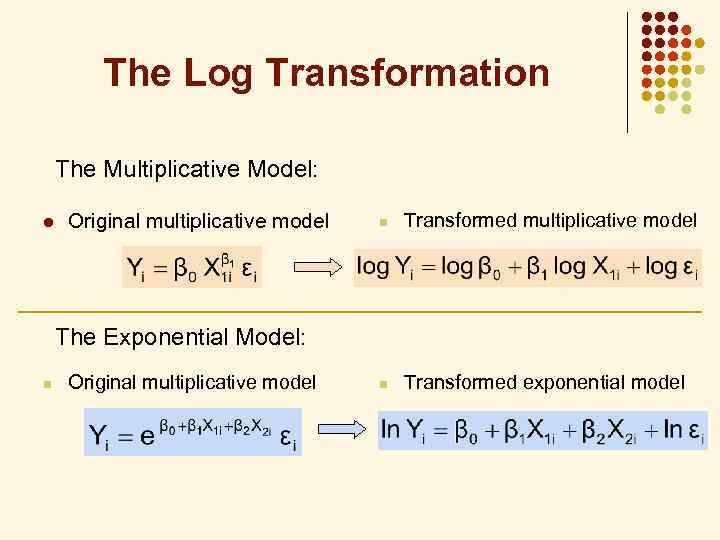The Log Transformation The Multiplicative Model: l Original multiplicative model n Transformed exponential model The Exponential Model: n Original multiplicative model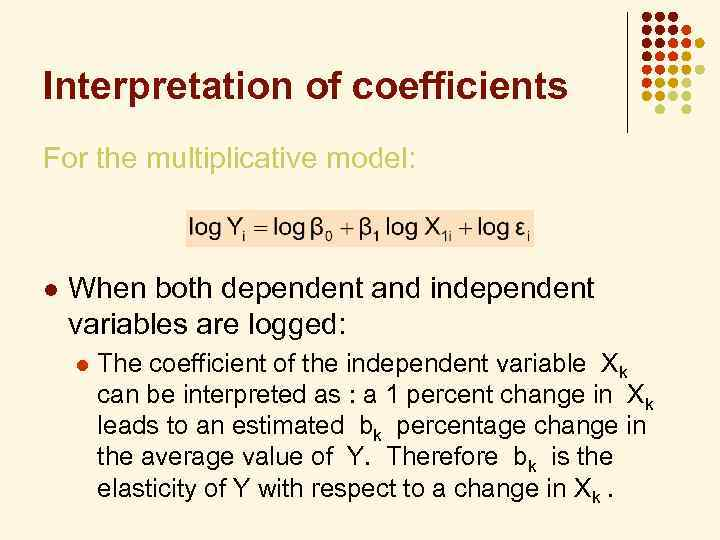Interpretation of coefficients For the multiplicative model: l When both dependent and independent variables are logged: l The coefficient of the independent variable Xk can be interpreted as : a 1 percent change in Xk leads to an estimated bk percentage change in the average value of Y. Therefore bk is the elasticity of Y with respect to a change in Xk.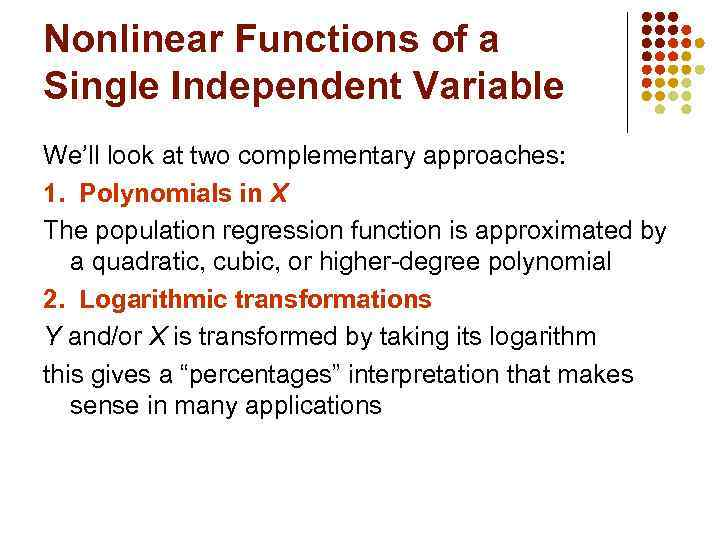Nonlinear Functions of a Single Independent Variable We’ll look at two complementary approaches: 1. Polynomials in X The population regression function is approximated by a quadratic, cubic, or higher-degree polynomial 2. Logarithmic transformations Y and/or X is transformed by taking its logarithm this gives a “percentages” interpretation that makes sense in many applications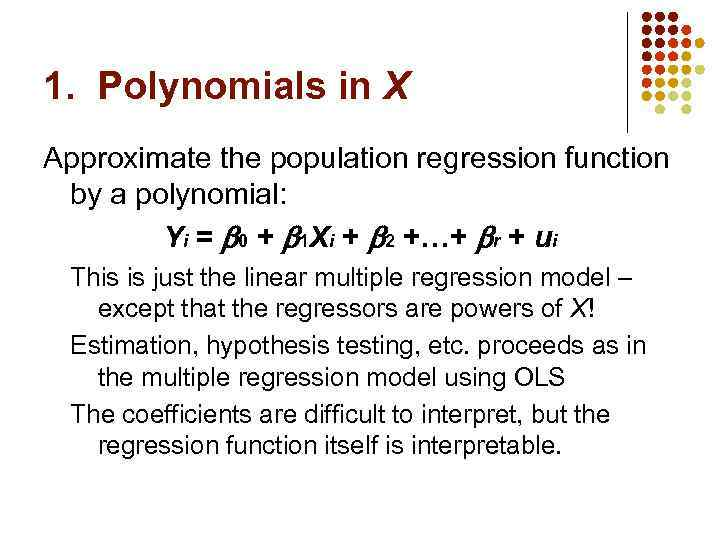1. Polynomials in X Approximate the population regression function by a polynomial: Yi = 0 + 1 Xi + 2 +…+ r + ui This is just the linear multiple regression model – except that the regressors are powers of X! Estimation, hypothesis testing, etc. proceeds as in the multiple regression model using OLS The coefficients are difficult to interpret, but the regression function itself is interpretable.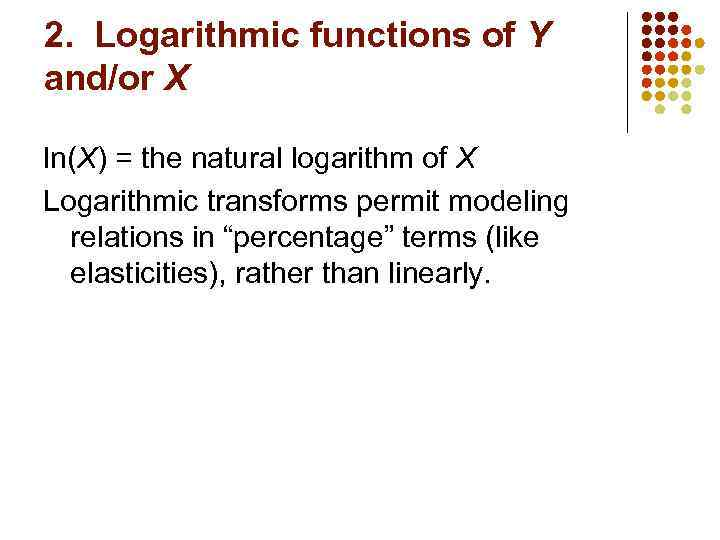2. Logarithmic functions of Y and/or X ln(X) = the natural logarithm of X Logarithmic transforms permit modeling relations in “percentage” terms (like elasticities), rather than linearly.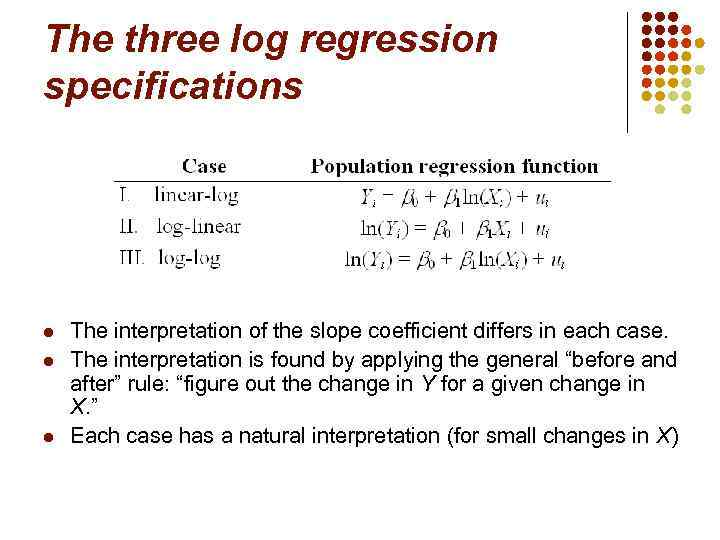The three log regression specifications l l l The interpretation of the slope coefficient differs in each case. The interpretation is found by applying the general “before and after” rule: “figure out the change in Y for a given change in X. ” Each case has a natural interpretation (for small changes in X)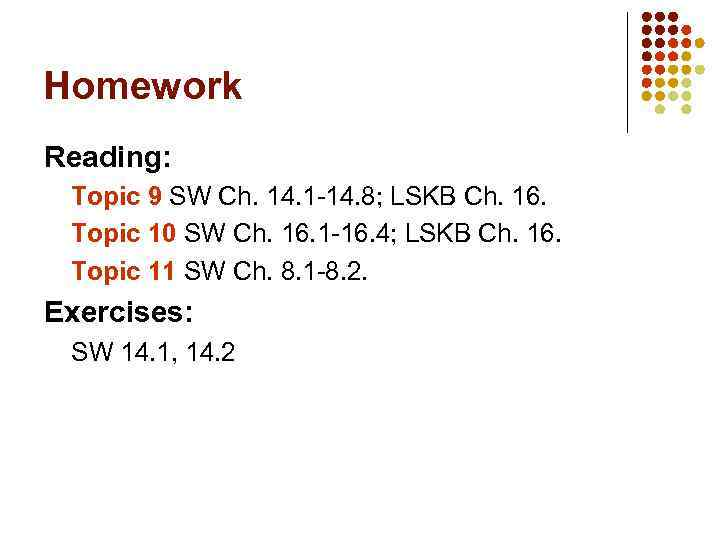Homework Reading: Topic 9 SW Ch. 14. 1 -14. 8; LSKB Ch. 16. Topic 10 SW Ch. 16. 1 -16. 4; LSKB Ch. 16. Topic 11 SW Ch. 8. 1 -8. 2. Exercises: SW 14. 1, 14. 2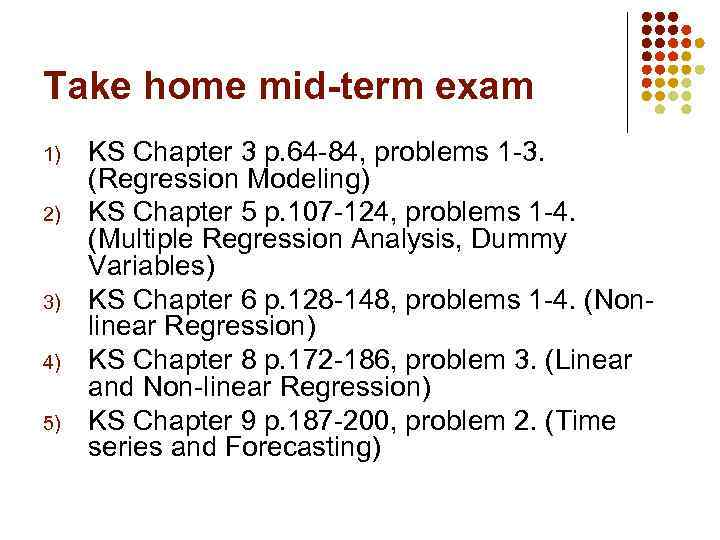Take home mid-term exam 1) 2) 3) 4) 5) KS Chapter 3 p. 64 -84, problems 1 -3. (Regression Modeling) KS Chapter 5 p. 107 -124, problems 1 -4. (Multiple Regression Analysis, Dummy Variables) KS Chapter 6 p. 128 -148, problems 1 -4. (Nonlinear Regression) KS Chapter 8 p. 172 -186, problem 3. (Linear and Non-linear Regression) KS Chapter 9 p. 187 -200, problem 2. (Time series and Forecasting)QUANTITATIVE METHODS OF BUSINESS RESEARCH Class 6. Topic 12. Categorical independent variables, interaction terms.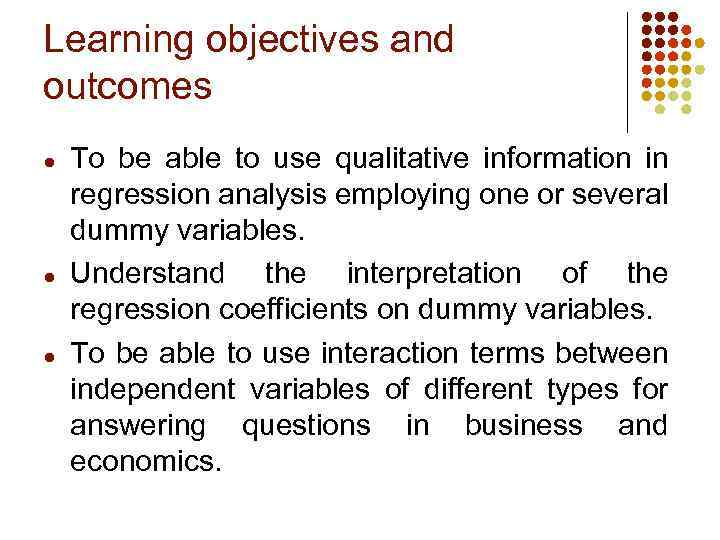Learning objectives and outcomes ● ● ● To be able to use qualitative information in regression analysis employing one or several dummy variables. Understand the interpretation of the regression coefficients on dummy variables. To be able to use interaction terms between independent variables of different types for answering questions in business and economics.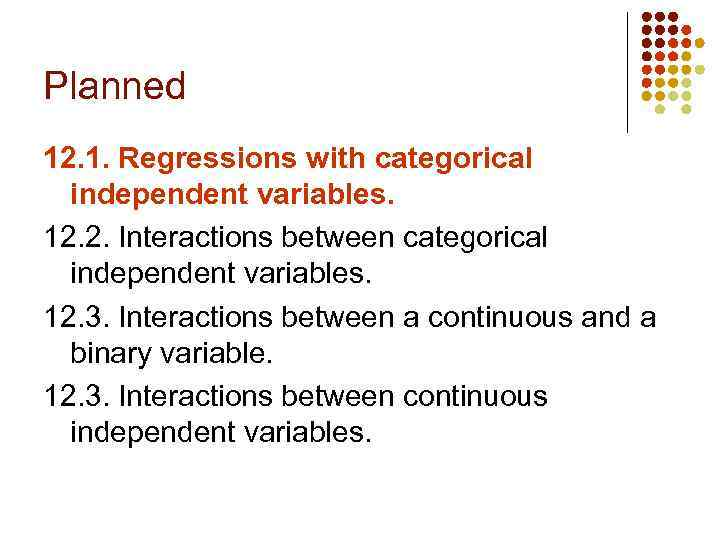Planned 12. 1. Regressions with categorical independent variables. 12. 2. Interactions between categorical independent variables. 12. 3. Interactions between a continuous and a binary variable. 12. 3. Interactions between continuous independent variables.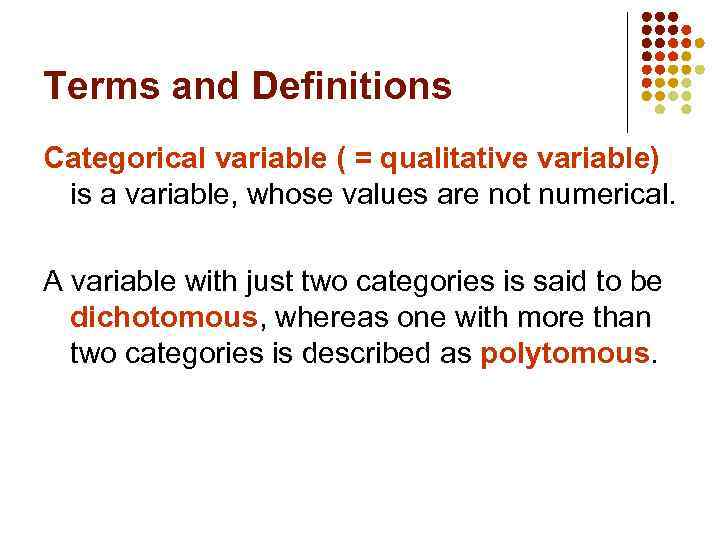Terms and Definitions Categorical variable ( = qualitative variable) is a variable, whose values are not numerical. A variable with just two categories is said to be dichotomous, whereas one with more than two categories is described as polytomous.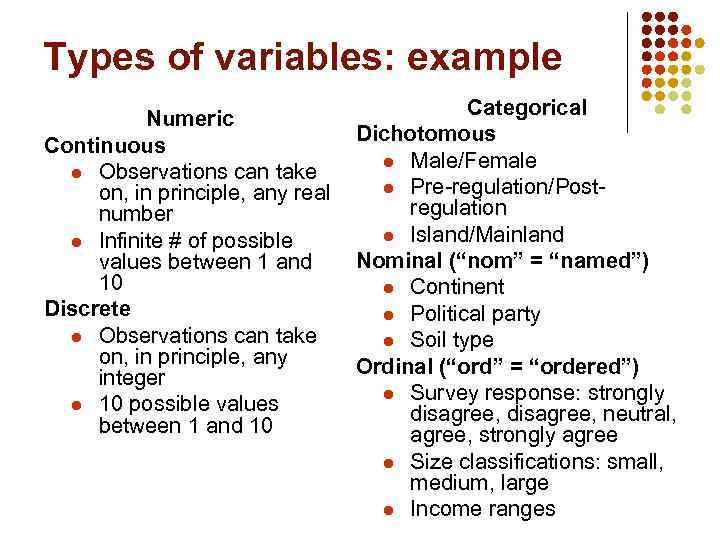Types of variables: example Categorical Numeric Dichotomous Continuous l Male/Female l Observations can take l Pre-regulation/Poston, in principle, any real regulation number l Island/Mainland l Infinite # of possible Nominal (“nom” = “named”) values between 1 and 10 l Continent Discrete l Political party l Observations can take l Soil type on, in principle, any Ordinal (“ord” = “ordered”) integer l Survey response: strongly l 10 possible values disagree, neutral, between 1 and 10 agree, strongly agree l Size classifications: small, medium, large l Income ranges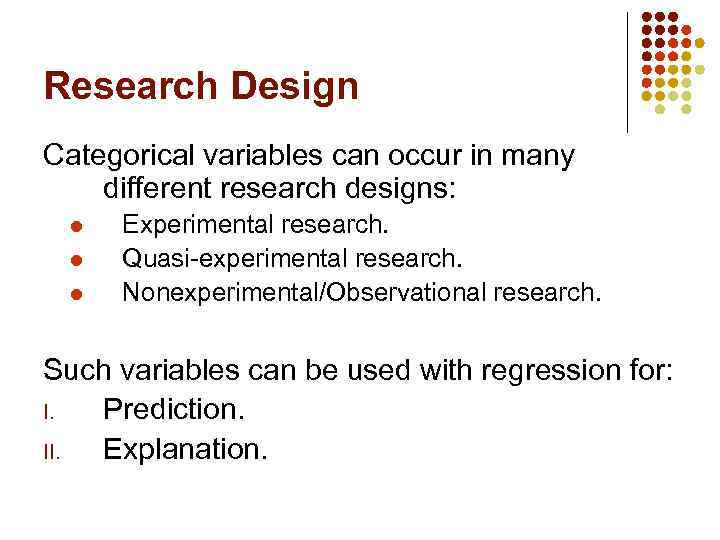Research Design Categorical variables can occur in many different research designs: l l l Experimental research. Quasi-experimental research. Nonexperimental/Observational research. Such variables can be used with regression for: I. Prediction. II. Explanation.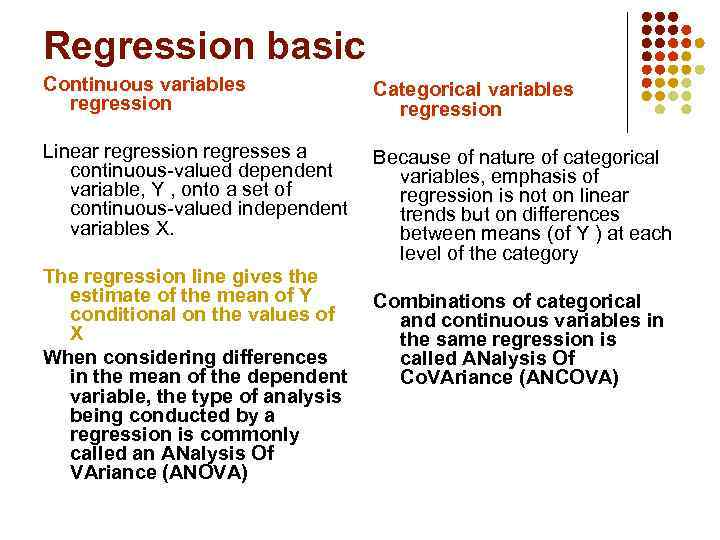Regression basic Continuous variables regression Categorical variables regression Linear regression regresses a Because of nature of categorical continuous-valued dependent variables, emphasis of variable, Y , onto a set of regression is not on linear continuous-valued independent trends but on differences variables X. between means (of Y ) at each level of the category The regression line gives the estimate of the mean of Y Combinations of categorical conditional on the values of and continuous variables in X the same regression is When considering differences called ANalysis Of in the mean of the dependent Co. VAriance (ANCOVA) variable, the type of analysis being conducted by a regression is commonly called an ANalysis Of VAriance (ANOVA)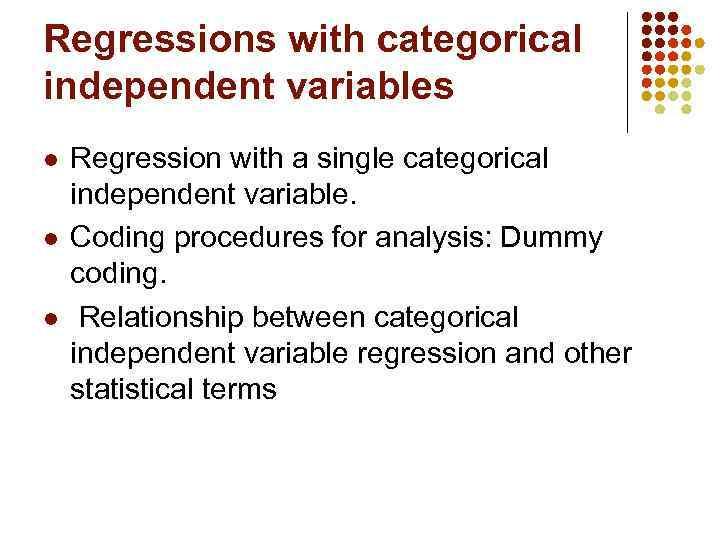Regressions with categorical independent variables l l l Regression with a single categorical independent variable. Coding procedures for analysis: Dummy coding. Relationship between categorical independent variable regression and other statistical terms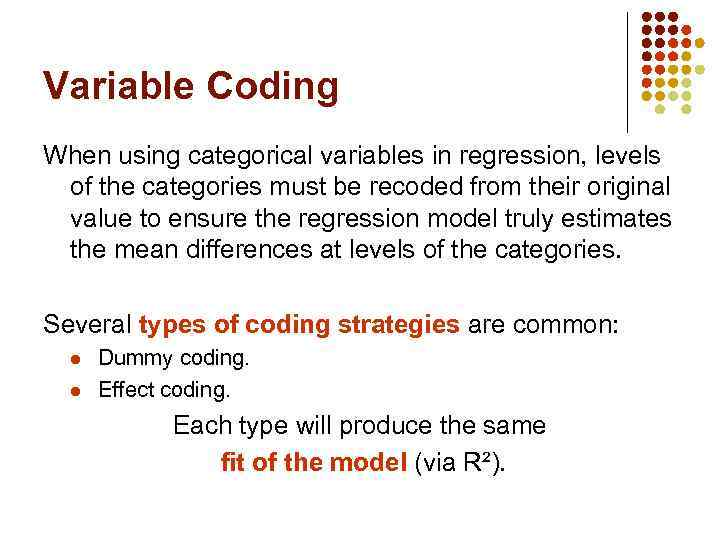Variable Coding When using categorical variables in regression, levels of the categories must be recoded from their original value to ensure the regression model truly estimates the mean differences at levels of the categories. Several types of coding strategies are common: l l Dummy coding. Effect coding. Each type will produce the same ﬁt of the model (via R²).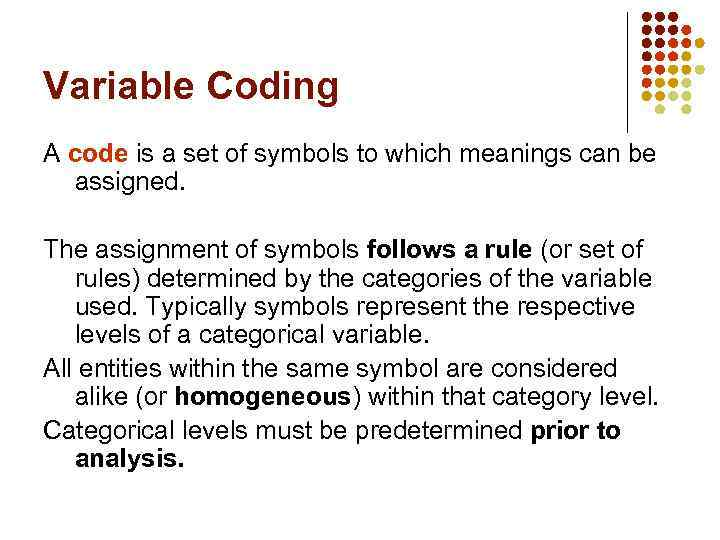Variable Coding A code is a set of symbols to which meanings can be assigned. The assignment of symbols follows a rule (or set of rules) determined by the categories of the variable used. Typically symbols represent the respective levels of a categorical variable. All entities within the same symbol are considered alike (or homogeneous) within that category level. Categorical levels must be predetermined prior to analysis.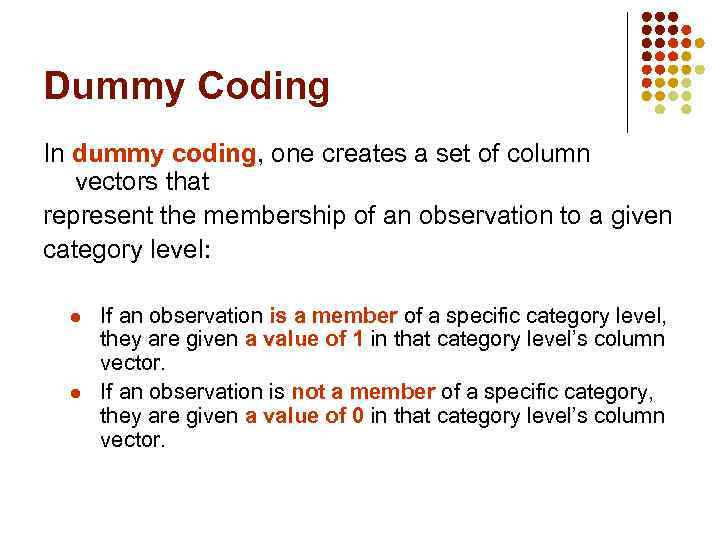Dummy Coding In dummy coding, one creates a set of column vectors that represent the membership of an observation to a given category level: l l If an observation is a member of a specific category level, they are given a value of 1 in that category level’s column vector. If an observation is not a member of a specific category, they are given a value of 0 in that category level’s column vector.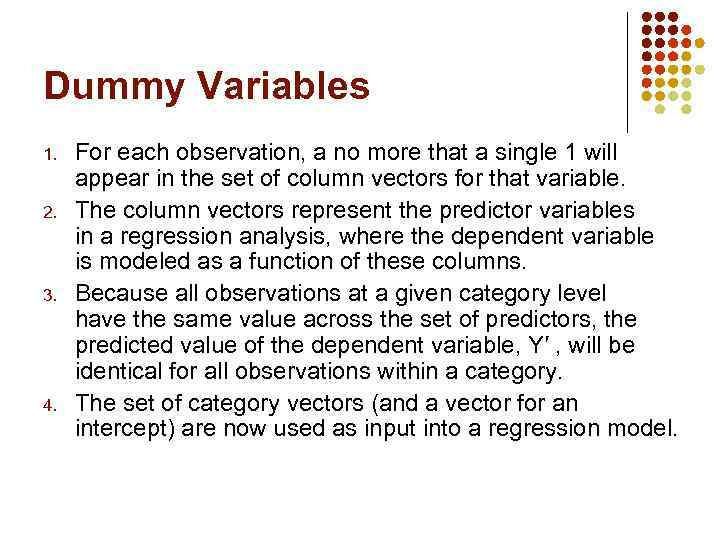Dummy Variables 1. 2. 3. 4. For each observation, a no more that a single 1 will appear in the set of column vectors for that variable. The column vectors represent the predictor variables in a regression analysis, where the dependent variable is modeled as a function of these columns. Because all observations at a given category level have the same value across the set of predictors, the predicted value of the dependent variable, Y′ , will be identical for all observations within a category. The set of category vectors (and a vector for an intercept) are now used as input into a regression model.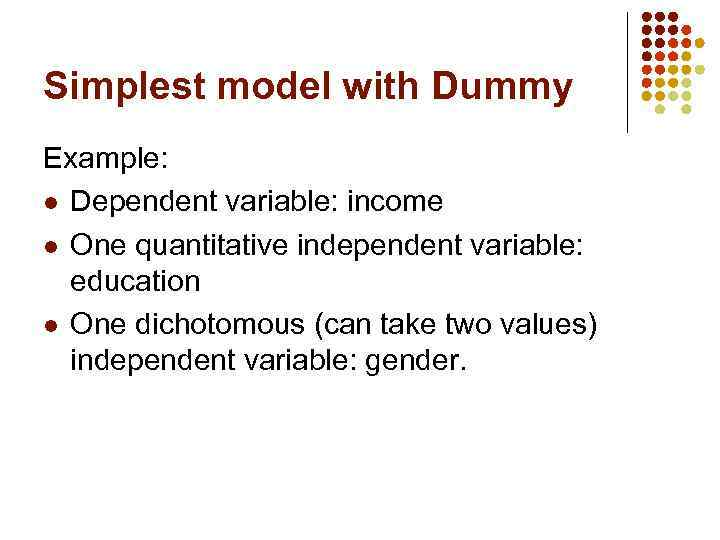Simplest model with Dummy Example: l Dependent variable: income l One quantitative independent variable: education l One dichotomous (can take two values) independent variable: gender.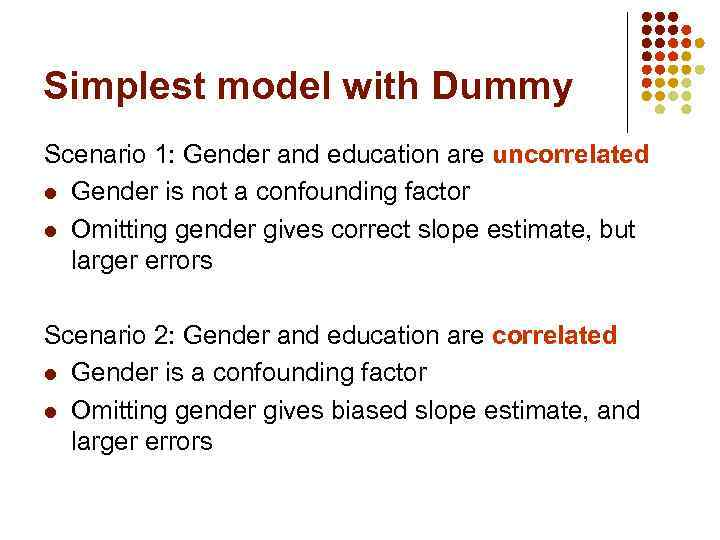Simplest model with Dummy Scenario 1: Gender and education are uncorrelated l Gender is not a confounding factor l Omitting gender gives correct slope estimate, but larger errors Scenario 2: Gender and education are correlated l Gender is a confounding factor l Omitting gender gives biased slope estimate, and larger errors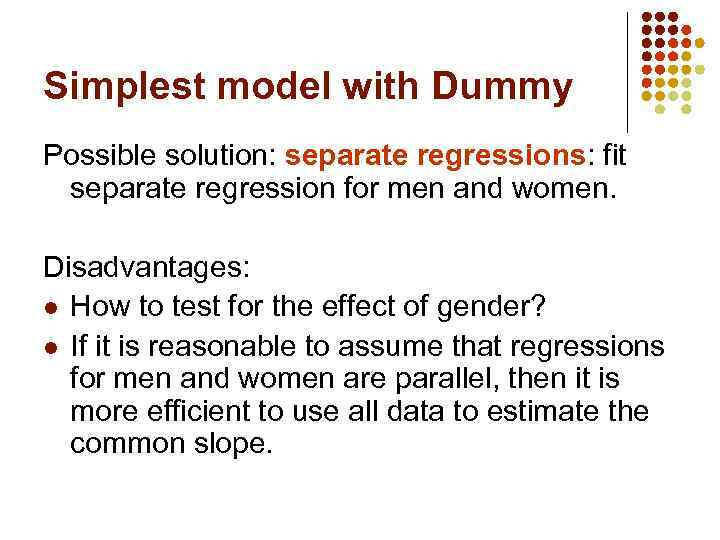Simplest model with Dummy Possible solution: separate regressions: fit separate regression for men and women. Disadvantages: l How to test for the effect of gender? l If it is reasonable to assume that regressions for men and women are parallel, then it is more efficient to use all data to estimate the common slope.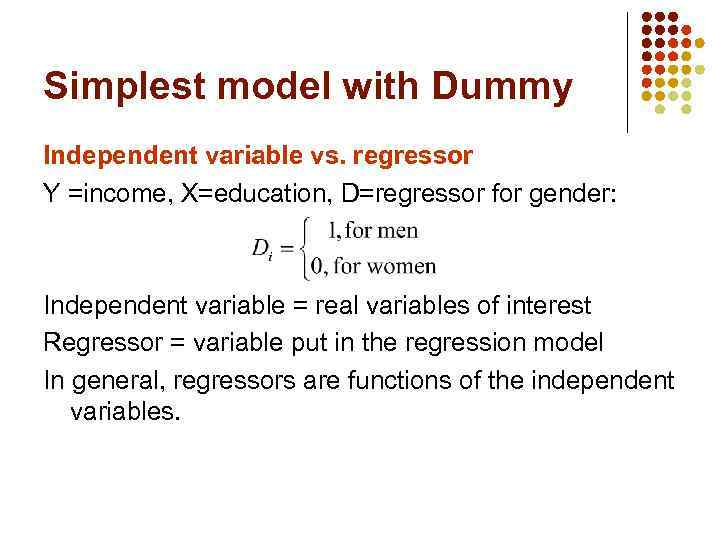Simplest model with Dummy Independent variable vs. regressor Y =income, X=education, D=regressor for gender: Independent variable = real variables of interest Regressor = variable put in the regression model In general, regressors are functions of the independent variables.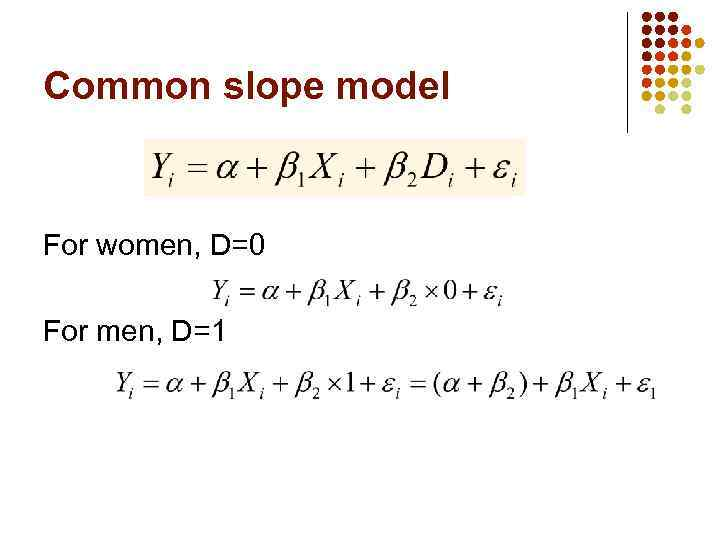Common slope model For women, D=0 For men, D=1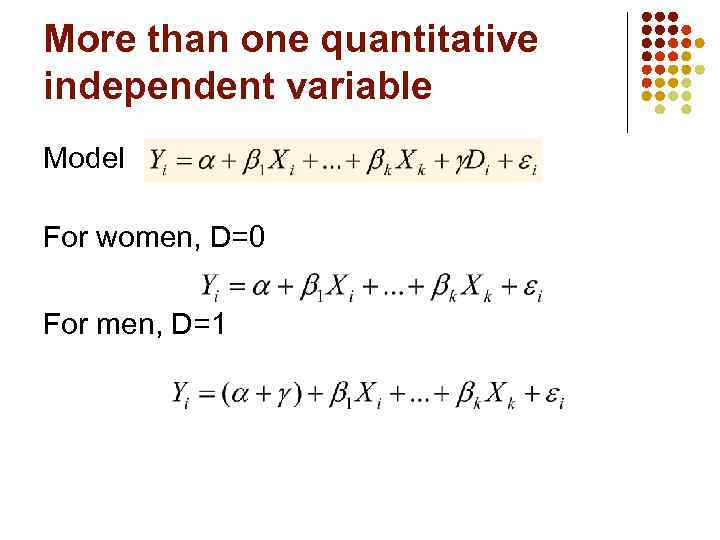More than one quantitative independent variable Model For women, D=0 For men, D=1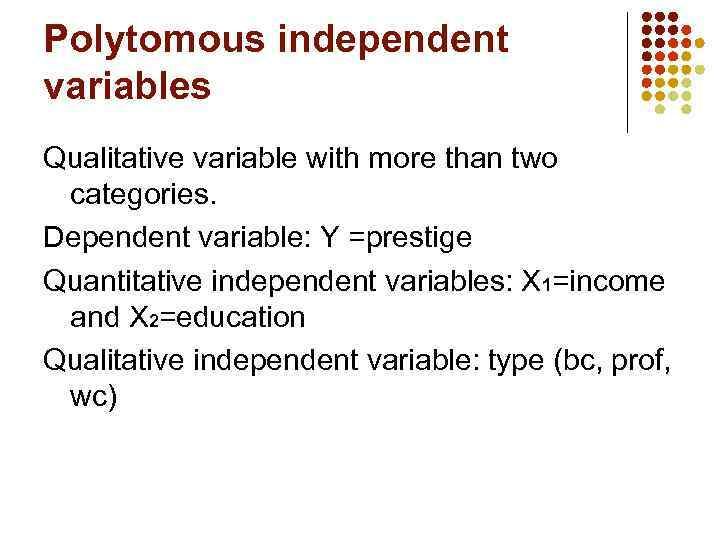Polytomous independent variables Qualitative variable with more than two categories. Dependent variable: Y =prestige Quantitative independent variables: X 1=income and X 2=education Qualitative independent variable: type (bc, prof, wc)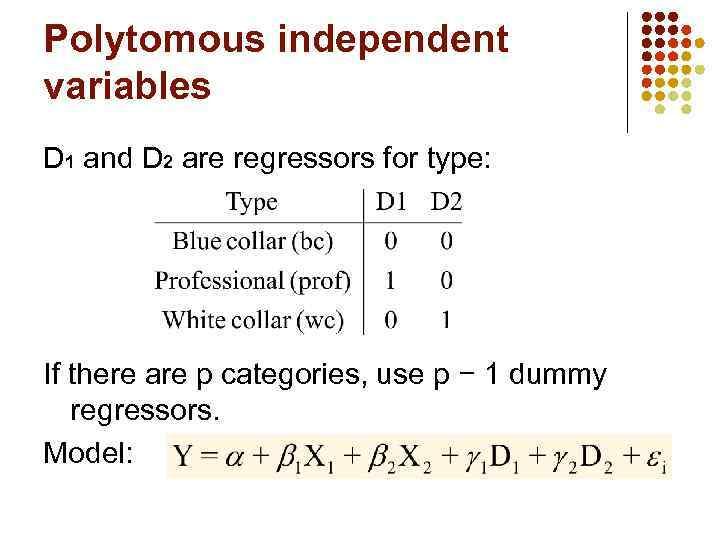Polytomous independent variables D 1 and D 2 are regressors for type: If there are p categories, use p − 1 dummy regressors. Model: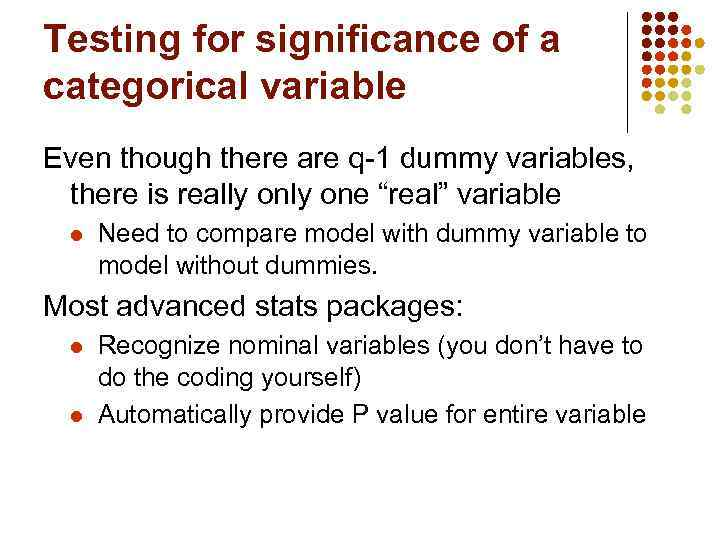Testing for significance of a categorical variable Even though there are q-1 dummy variables, there is really one “real” variable l Need to compare model with dummy variable to model without dummies. Most advanced stats packages: l l Recognize nominal variables (you don’t have to do the coding yourself) Automatically provide P value for entire variable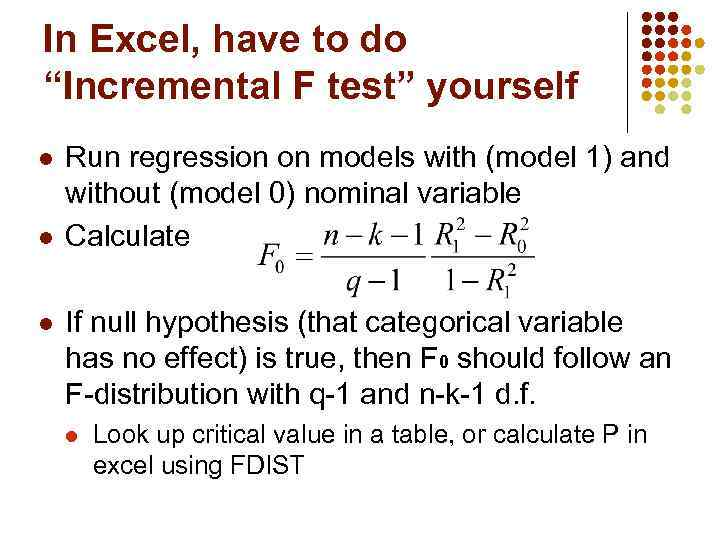In Excel, have to do “Incremental F test” yourself l l l Run regression on models with (model 1) and without (model 0) nominal variable Calculate If null hypothesis (that categorical variable has no effect) is true, then F 0 should follow an F-distribution with q-1 and n-k-1 d. f. l Look up critical value in a table, or calculate P in excel using FDIST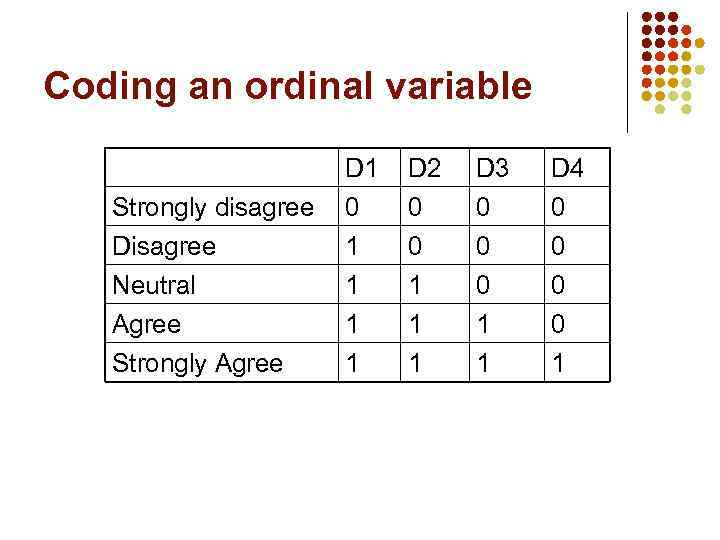Coding an ordinal variable Strongly disagree Disagree Neutral D 1 0 1 1 D 2 0 0 1 D 3 0 0 0 D 4 0 0 0 Agree Strongly Agree 1 1 1 0 1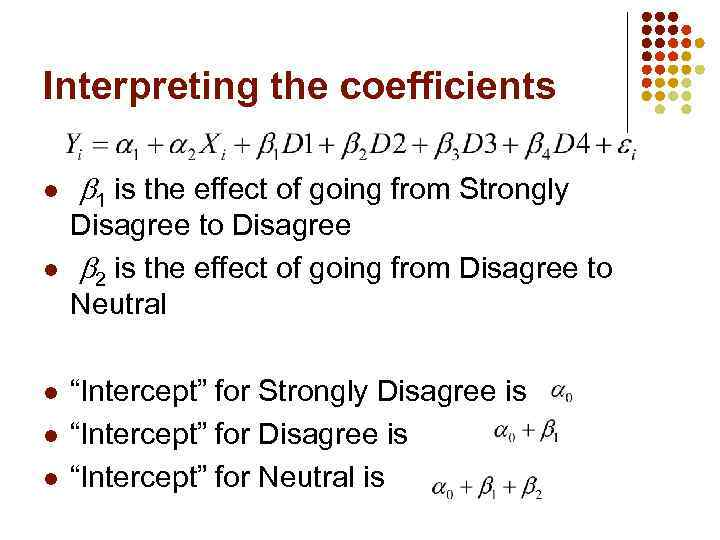Interpreting the coefficients l l l 1 is the effect of going from Strongly Disagree to Disagree 2 is the effect of going from Disagree to Neutral “Intercept” for Strongly Disagree is “Intercept” for Neutral is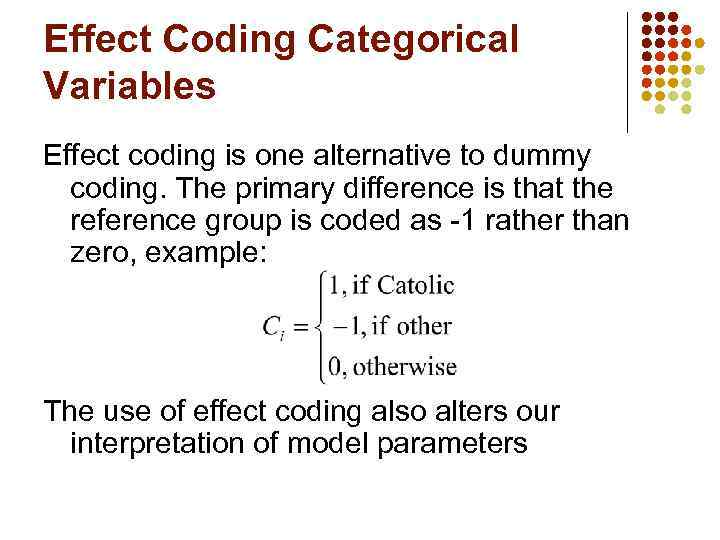Effect Coding Categorical Variables Effect coding is one alternative to dummy coding. The primary difference is that the reference group is coded as -1 rather than zero, example: The use of effect coding also alters our interpretation of model parametersInterpreting Regression Coefficients with Effect Coding l l The intercept is now interpreted as the grand mean, or average of averages. The slopes now compare group averages to the grand mean. Although the coding is different you will make the same statistical inferences. Effect coding is a good alternative when you want to have a simple interpretation for Yintercept.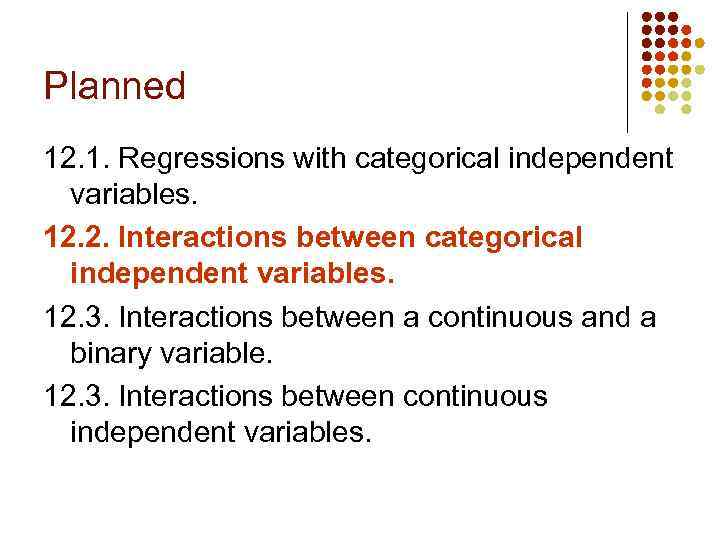Planned 12. 1. Regressions with categorical independent variables. 12. 2. Interactions between categorical independent variables. 12. 3. Interactions between a continuous and a binary variable. 12. 3. Interactions between continuous independent variables.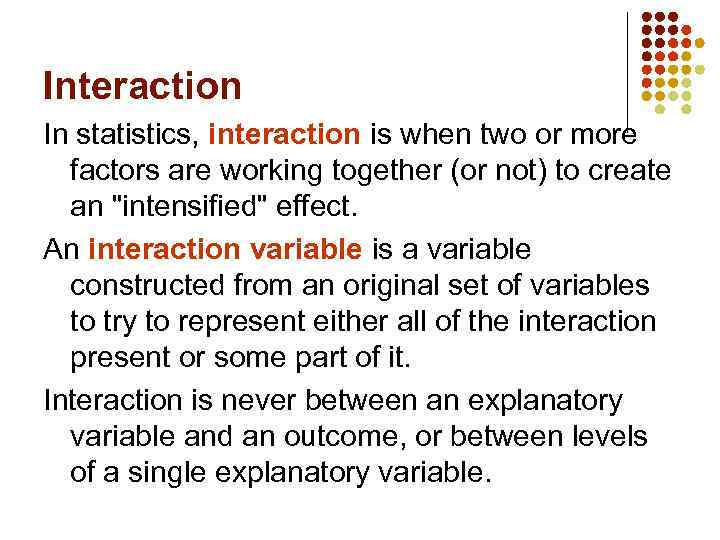Interaction In statistics, interaction is when two or more factors are working together (or not) to create an "intensified" effect. An interaction variable is a variable constructed from an original set of variables to try to represent either all of the interaction present or some part of it. Interaction is never between an explanatory variable and an outcome, or between levels of a single explanatory variable.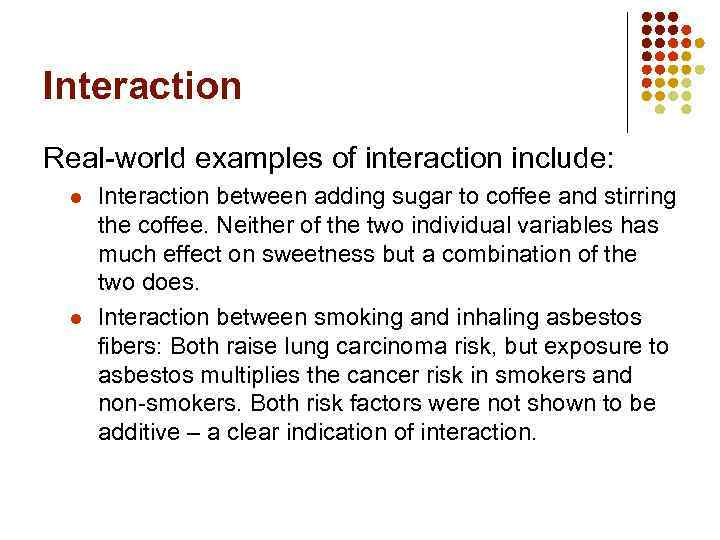Interaction Real-world examples of interaction include: l l Interaction between adding sugar to coffee and stirring the coffee. Neither of the two individual variables has much effect on sweetness but a combination of the two does. Interaction between smoking and inhaling asbestos fibers: Both raise lung carcinoma risk, but exposure to asbestos multiplies the cancer risk in smokers and non-smokers. Both risk factors were not shown to be additive – a clear indication of interaction.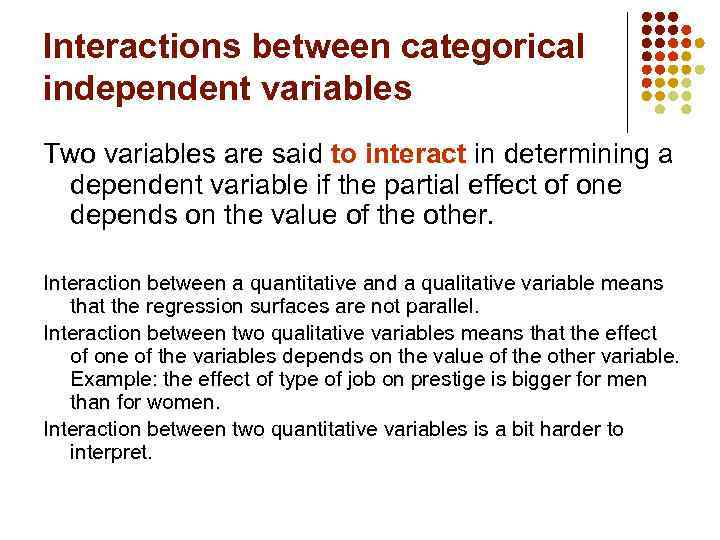Interactions between categorical independent variables Two variables are said to interact in determining a dependent variable if the partial effect of one depends on the value of the other. Interaction between a quantitative and a qualitative variable means that the regression surfaces are not parallel. Interaction between two qualitative variables means that the effect of one of the variables depends on the value of the other variable. Example: the effect of type of job on prestige is bigger for men than for women. Interaction between two quantitative variables is a bit harder to interpret.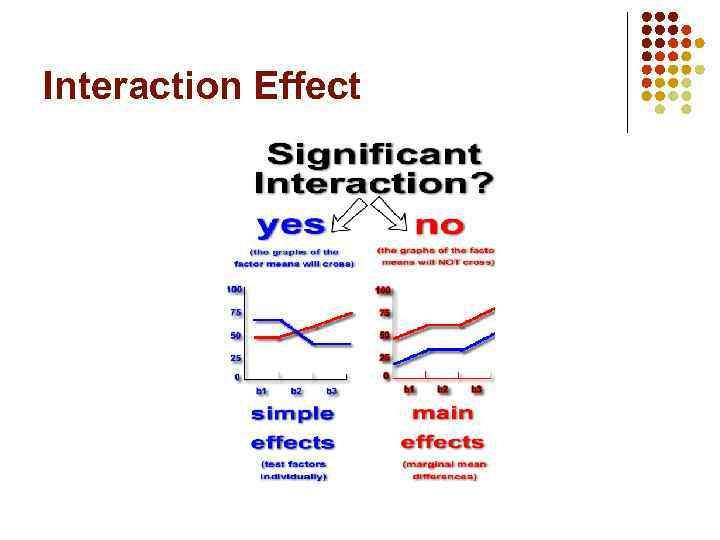Interaction Effect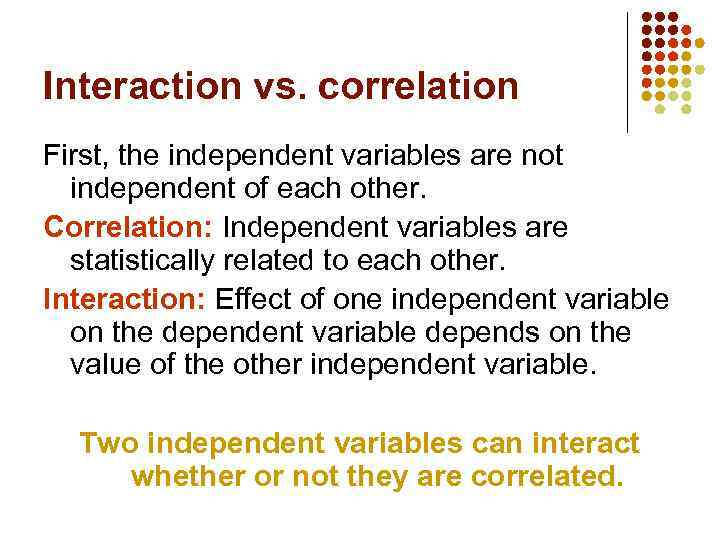Interaction vs. correlation First, the independent variables are not independent of each other. Correlation: Independent variables are statistically related to each other. Interaction: Effect of one independent variable on the dependent variable depends on the value of the other independent variable. Two independent variables can interact whether or not they are correlated.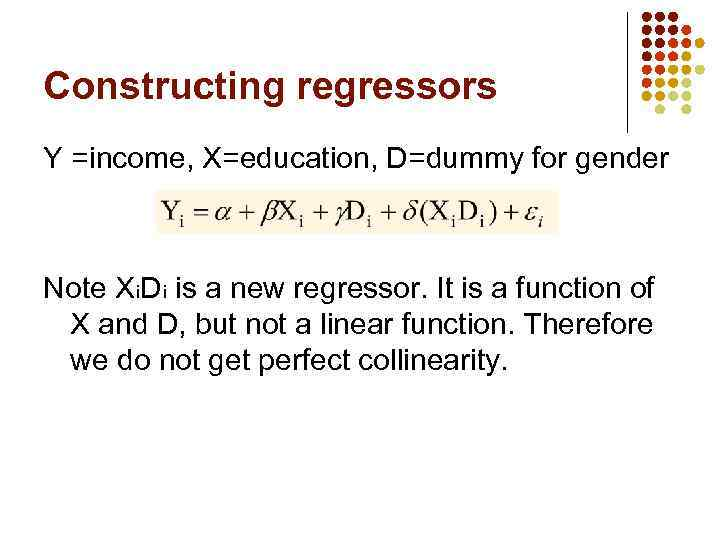Constructing regressors Y =income, X=education, D=dummy for gender Note Xi. Di is a new regressor. It is a function of X and D, but not a linear function. Therefore we do not get perfect collinearity.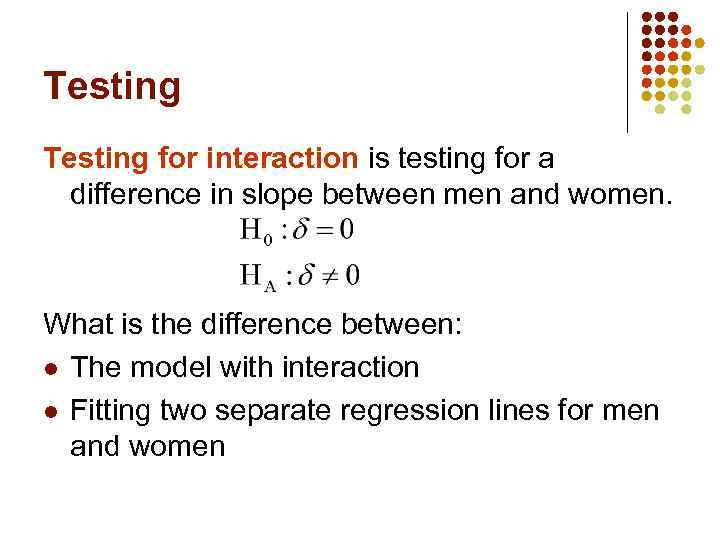Testing for interaction is testing for a difference in slope between men and women. What is the diﬀerence between: l The model with interaction l Fitting two separate regression lines for men and women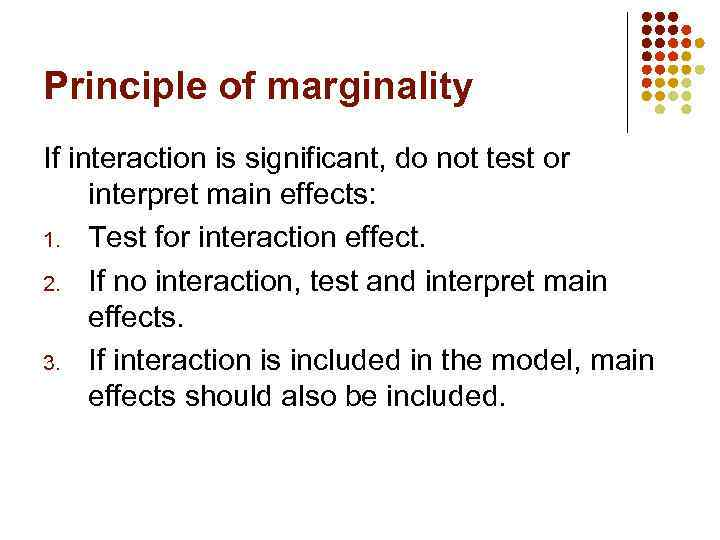Principle of marginality If interaction is significant, do not test or interpret main effects: 1. Test for interaction effect. 2. If no interaction, test and interpret main effects. 3. If interaction is included in the model, main effects should also be included.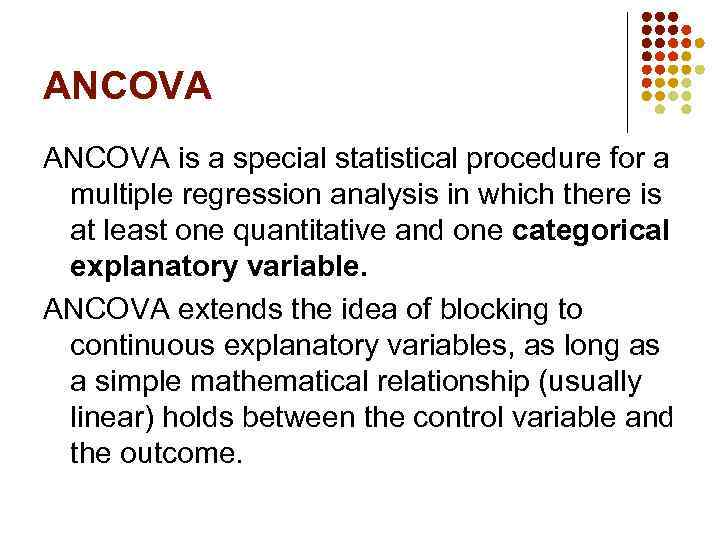ANCOVA is a special statistical procedure for a multiple regression analysis in which there is at least one quantitative and one categorical explanatory variable. ANCOVA extends the idea of blocking to continuous explanatory variables, as long as a simple mathematical relationship (usually linear) holds between the control variable and the outcome.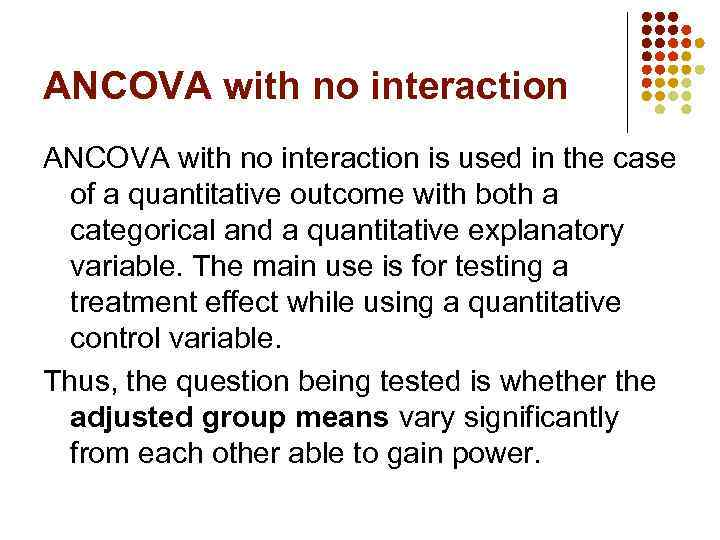ANCOVA with no interaction is used in the case of a quantitative outcome with both a categorical and a quantitative explanatory variable. The main use is for testing a treatment effect while using a quantitative control variable. Thus, the question being tested is whether the adjusted group means vary significantly from each other able to gain power.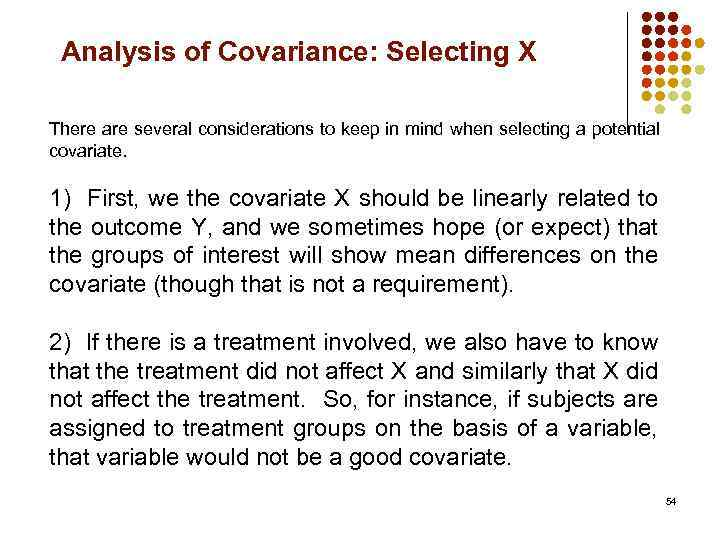Analysis of Covariance: Selecting X There are several considerations to keep in mind when selecting a potential covariate. 1) First, we the covariate X should be linearly related to the outcome Y, and we sometimes hope (or expect) that the groups of interest will show mean differences on the covariate (though that is not a requirement). 2) If there is a treatment involved, we also have to know that the treatment did not affect X and similarly that X did not affect the treatment. So, for instance, if subjects are assigned to treatment groups on the basis of a variable, that variable would not be a good covariate. 54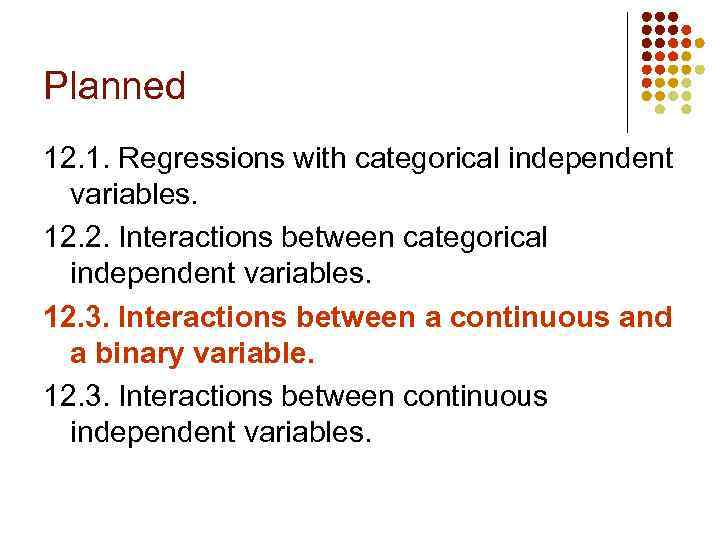Planned 12. 1. Regressions with categorical independent variables. 12. 2. Interactions between categorical independent variables. 12. 3. Interactions between a continuous and a binary variable. 12. 3. Interactions between continuous independent variables.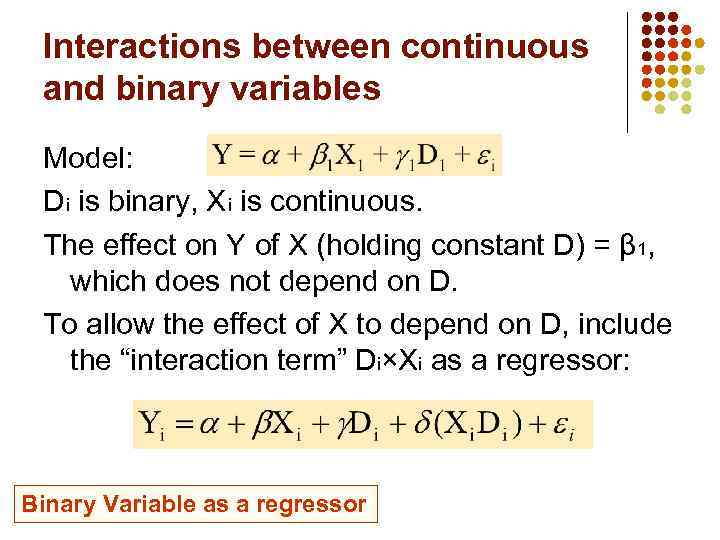Interactions between continuous and binary variables Model: Di is binary, Xi is continuous. The effect on Y of X (holding constant D) = β 1, which does not depend on D. To allow the effect of X to depend on D, include the “interaction term” Di×Xi as a regressor: Binary Variable as a regressor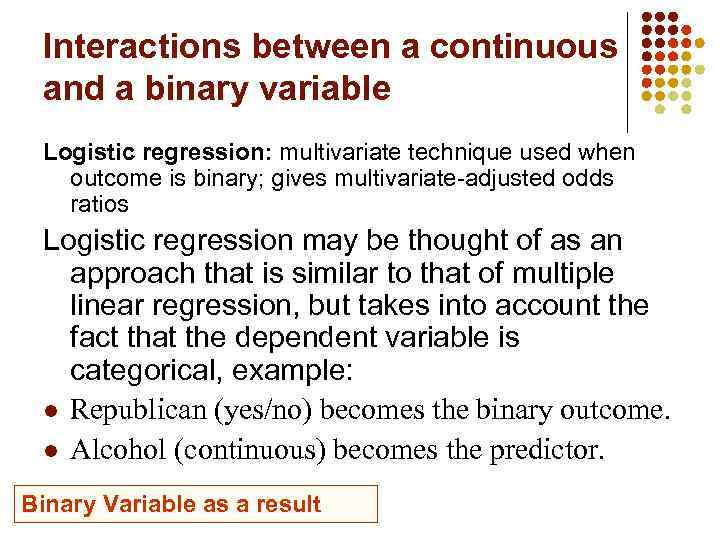Interactions between a continuous and a binary variable Logistic regression: multivariate technique used when outcome is binary; gives multivariate-adjusted odds ratios Logistic regression may be thought of as an approach that is similar to that of multiple linear regression, but takes into account the fact that the dependent variable is categorical, example: l Republican (yes/no) becomes the binary outcome. l Alcohol (continuous) becomes the predictor. Binary Variable as a result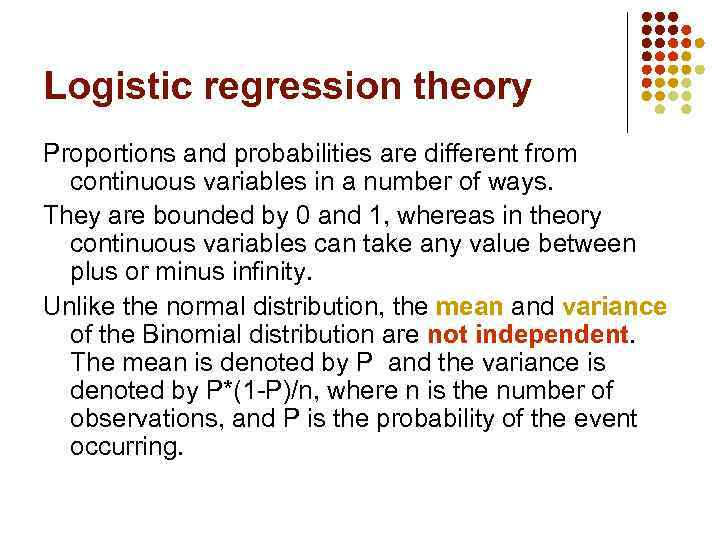Logistic regression theory Proportions and probabilities are different from continuous variables in a number of ways. They are bounded by 0 and 1, whereas in theory continuous variables can take any value between plus or minus infinity. Unlike the normal distribution, the mean and variance of the Binomial distribution are not independent. The mean is denoted by P and the variance is denoted by P*(1 -P)/n, where n is the number of observations, and P is the probability of the event occurring.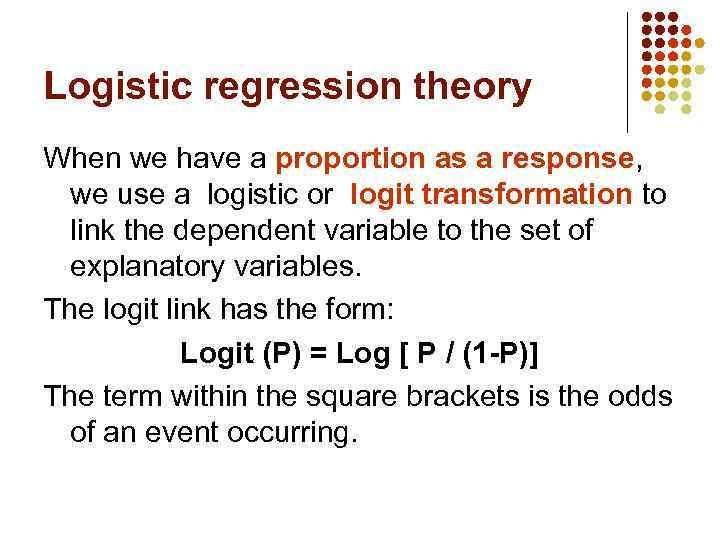Logistic regression theory When we have a proportion as a response, we use a logistic or logit transformation to link the dependent variable to the set of explanatory variables. The logit link has the form: Logit (P) = Log [ P / (1 -P)] The term within the square brackets is the odds of an event occurring.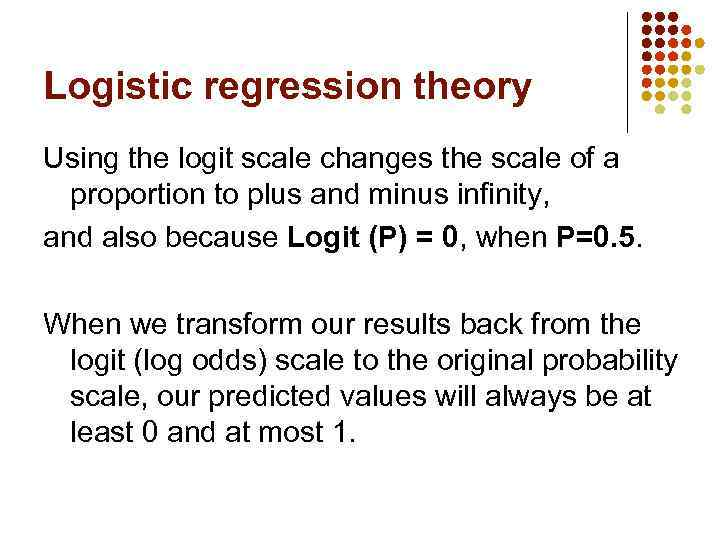Logistic regression theory Using the logit scale changes the scale of a proportion to plus and minus infinity, and also because Logit (P) = 0, when P=0. 5. When we transform our results back from the logit (log odds) scale to the original probability scale, our predicted values will always be at least 0 and at most 1.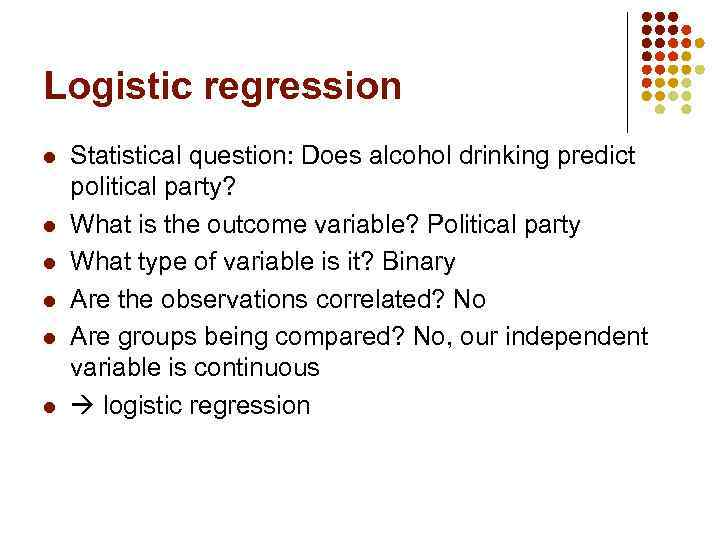Logistic regression l l l Statistical question: Does alcohol drinking predict political party? What is the outcome variable? Political party What type of variable is it? Binary Are the observations correlated? No Are groups being compared? No, our independent variable is continuous logistic regression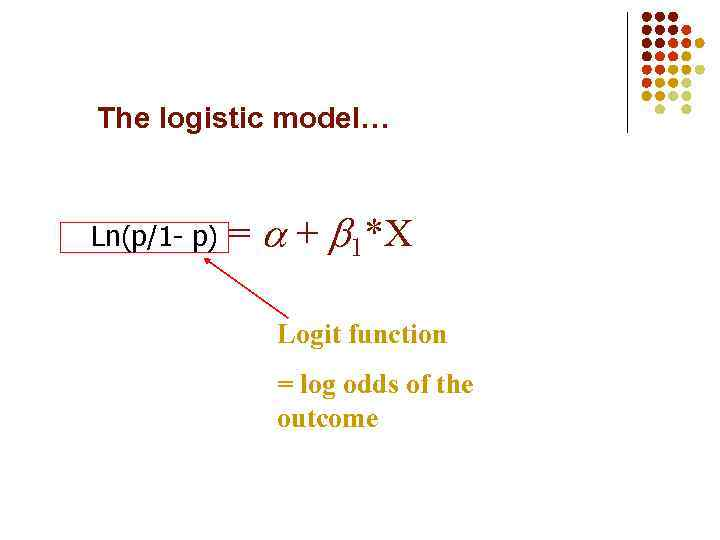The logistic model… Ln(p/1 - p) = + 1*X Logit function = log odds of the outcome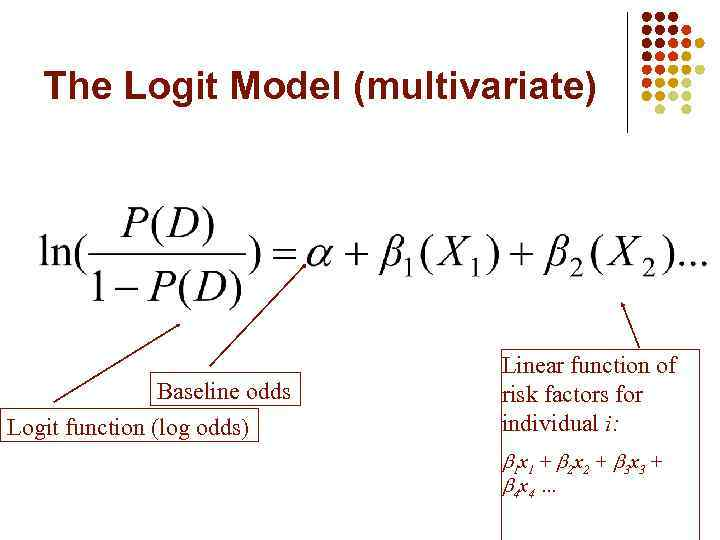The Logit Model (multivariate) Baseline odds Logit function (log odds) Linear function of risk factors for individual i: 1 x 1 + 2 x 2 + 3 x 3 + 4 x 4 …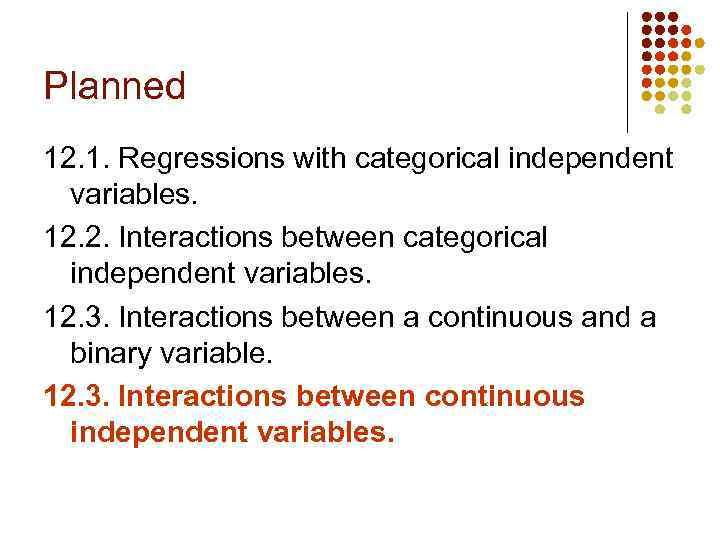Planned 12. 1. Regressions with categorical independent variables. 12. 2. Interactions between categorical independent variables. 12. 3. Interactions between a continuous and a binary variable. 12. 3. Interactions between continuous independent variables.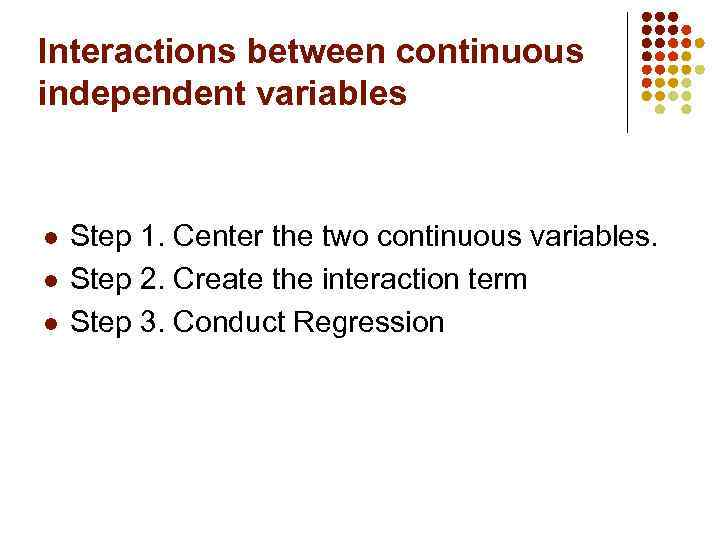Interactions between continuous independent variables l l l Step 1. Center the two continuous variables. Step 2. Create the interaction term Step 3. Conduct Regression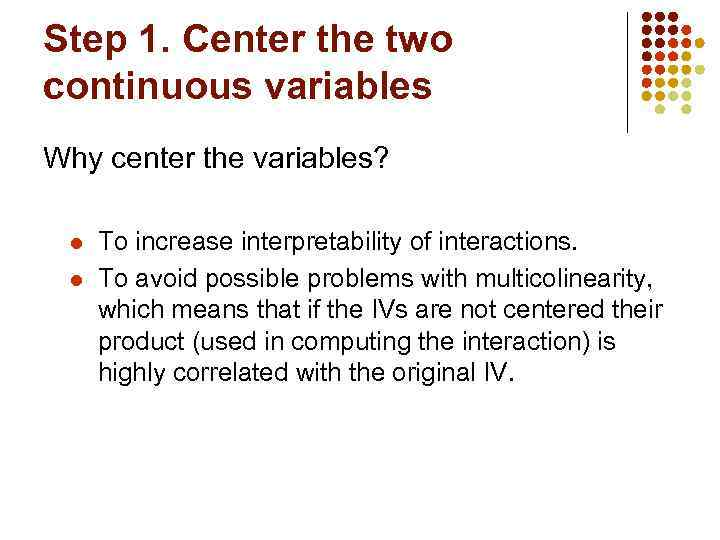Step 1. Center the two continuous variables Why center the variables? l l To increase interpretability of interactions. To avoid possible problems with multicolinearity, which means that if the IVs are not centered their product (used in computing the interaction) is highly correlated with the original IV.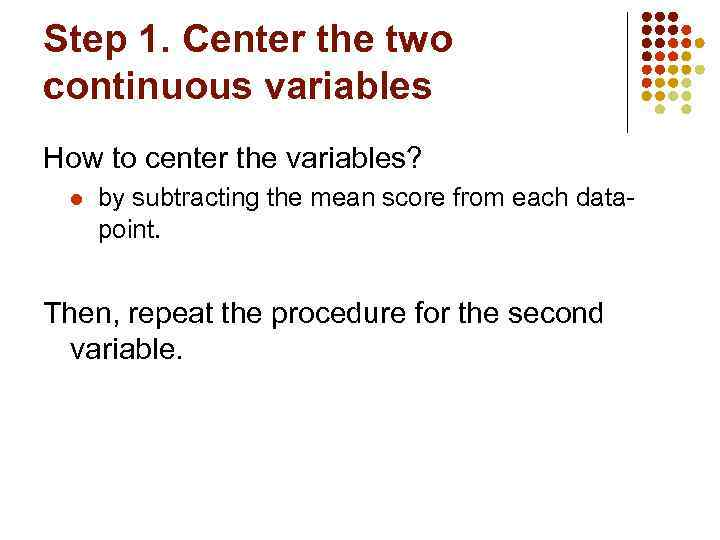Step 1. Center the two continuous variables How to center the variables? l by subtracting the mean score from each datapoint. Then, repeat the procedure for the second variable.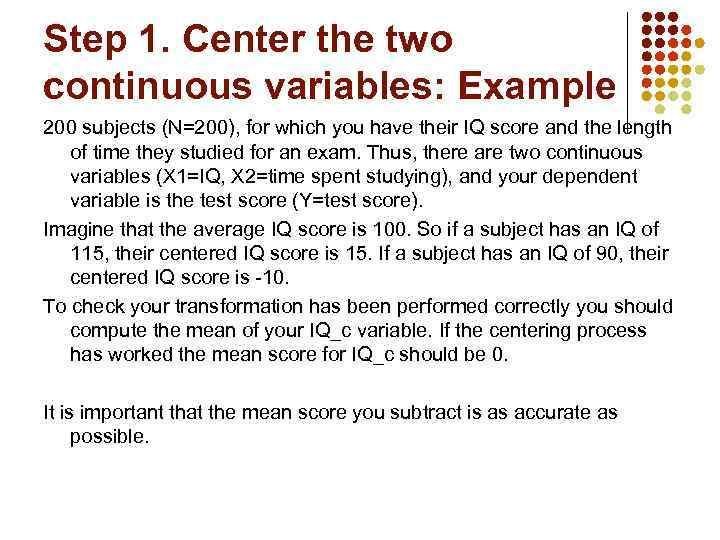Step 1. Center the two continuous variables: Example 200 subjects (N=200), for which you have their IQ score and the length of time they studied for an exam. Thus, there are two continuous variables (X 1=IQ, X 2=time spent studying), and your dependent variable is the test score (Y=test score). Imagine that the average IQ score is 100. So if a subject has an IQ of 115, their centered IQ score is 15. If a subject has an IQ of 90, their centered IQ score is -10. To check your transformation has been performed correctly you should compute the mean of your IQ_c variable. If the centering process has worked the mean score for IQ_c should be 0. It is important that the mean score you subtract is as accurate as possible.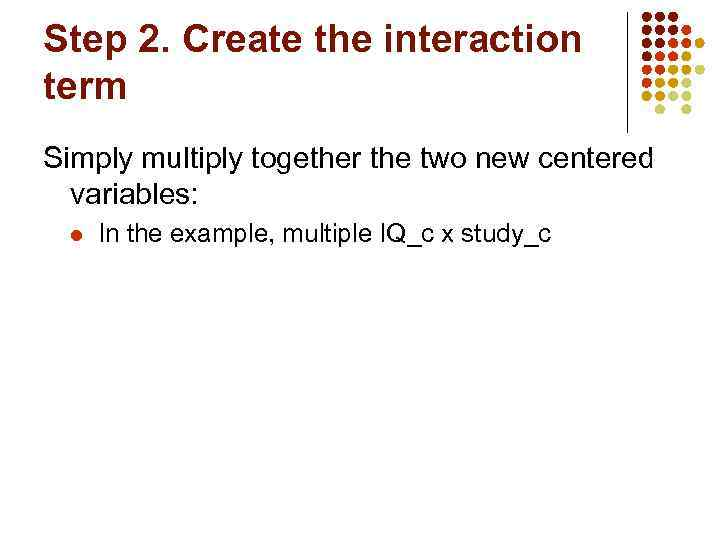Step 2. Create the interaction term Simply multiply together the two new centered variables: l In the example, multiple IQ_c x study_c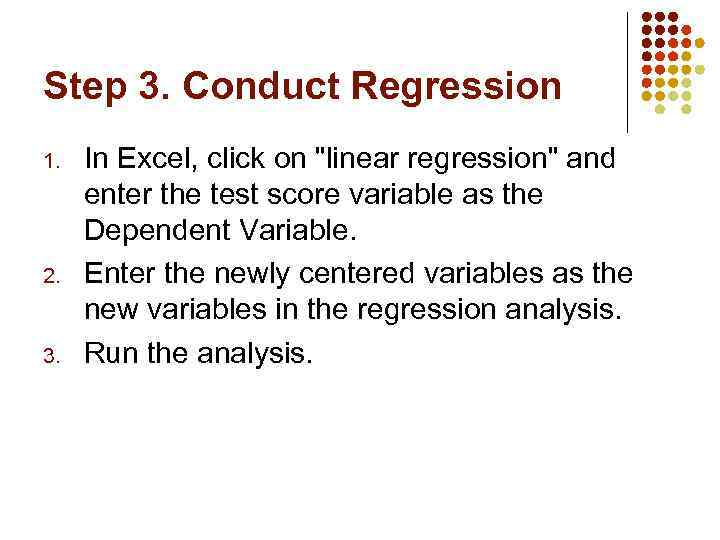Step 3. Conduct Regression 1. 2. 3. In Excel, click on "linear regression" and enter the test score variable as the Dependent Variable. Enter the newly centered variables as the new variables in the regression analysis. Run the analysis.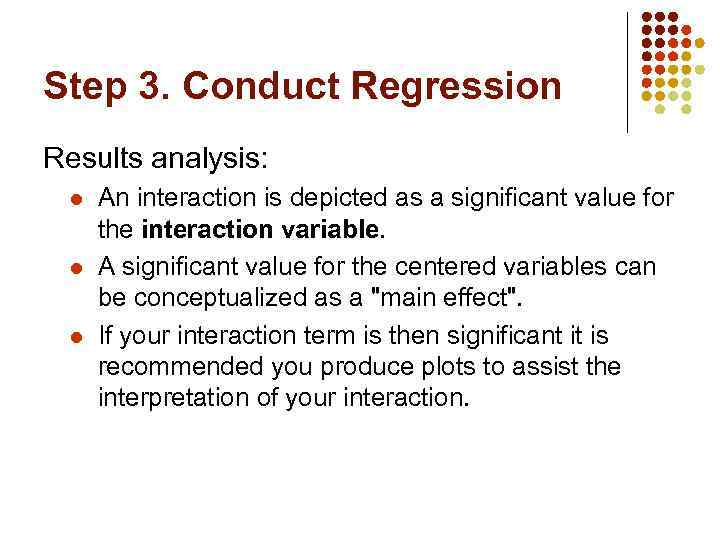Step 3. Conduct Regression Results analysis: l l l An interaction is depicted as a significant value for the interaction variable. A significant value for the centered variables can be conceptualized as a "main effect". If your interaction term is then significant it is recommended you produce plots to assist the interpretation of your interaction.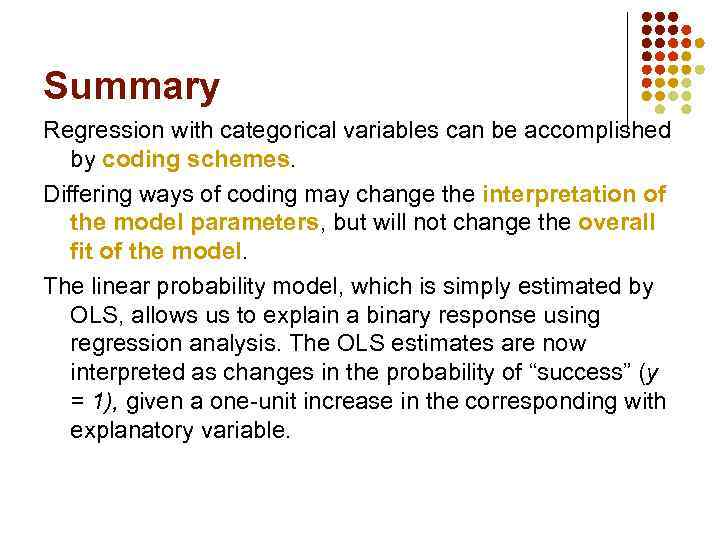Summary Regression with categorical variables can be accomplished by coding schemes. Differing ways of coding may change the interpretation of the model parameters, but will not change the overall ﬁt of the model. The linear probability model, which is simply estimated by OLS, allows us to explain a binary response using regression analysis. The OLS estimates are now interpreted as changes in the probability of “success” (y = 1), given a one-unit increase in the corresponding with explanatory variable.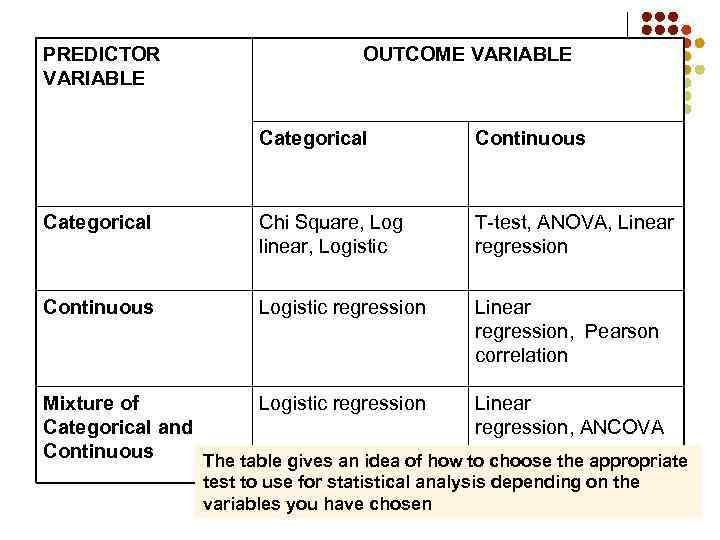PREDICTOR VARIABLE OUTCOME VARIABLE Categorical Continuous Categorical Chi Square, Log linear, Logistic T-test, ANOVA, Linear regression Continuous Logistic regression Linear regression, Pearson correlation Mixture of Logistic regression Linear Categorical and regression, ANCOVA Continuous The table gives an idea of how to choose the appropriate test to use for statistical analysis depending on the variables you have chosen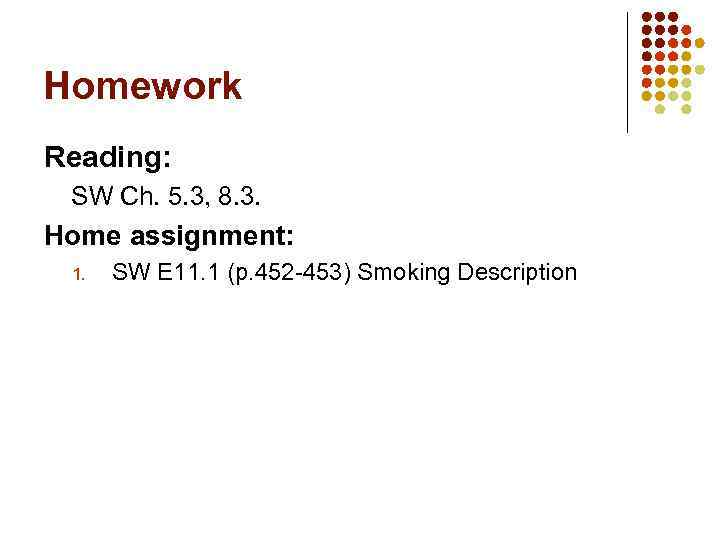Homework Reading: SW Ch. 5. 3, 8. 3. Home assignment: 1. SW E 11. 1 (p. 452 -453) Smoking Description